# Neatware Company

An ISV for Mobile, Cloud, and Video Technologies and Software.

GLSL Introduction

By Chang Li, Draft Version 0.5, March 20, 2013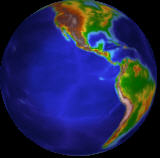OpenGL Shading Language (GLSL), a programming language for Graphic Processing Unit (GPU) in OpenGL platform, supports shader construction with C-like syntax, types, expressions, statements, and functions.

Long time ago, Apple's RenderMan was a popular shading language that was used to generate cinematic effects with CPU in render farms. Late, Microsoft's High-Level Shading Languages (HLSL) and OpenGL's OpenGL Shading Language (GLSL) shading languages have been developed for real-time shaders on GPU. OpenGL 1.5 to 4.0 and OpenGL ES started to include GLSL as a standard component. These high-level languages accelerated the shader development which plays a key role in game development.

To build a complete shader, GLSL must work along with a host programming language such as C/C++ along with OpenGL library. GLSL is widely used at the effect programming of games on ARM architecture like iOS, Android, as well as Linux platforms. This instruction below is pay more attention on OpenGL ES 2.0 and plus because of it has been widely used in mobile applications.

## Keywords

 Control: if, else, do, for, while, break, continue, return, discard Spec: const, attribute, uniform, varying Type: float, int, bool, true, false, void, lowp, mediump, highp, precision, invariant {vec2, vec3, vec4}, {ivec2, ivec3, ivec4}, {bvec2, bvec3, bvec4}, {mat2, mat3, mat4} Structure: struct, sampler1D, sampler2D, samplerCube Build-in: Attributes: gl_Vertex, gl_Normal, gl_Color, gl_MultiTexCord[#] Uniforms: gl_ModelViewMatrix, gl_ModelViewProjectionMatrix, gl_NormalMatrix Varyings: gl_FragColor, gl_BackColor, gl_TexCoord[#]

Note: The keyword list of OpenGL is very short. It is easy to programming. The strikeout keywords are used in special applications not be suggested to use.

## The Simplest Example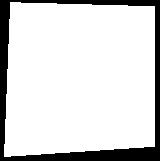A shader is usually consist of a vertex shader and a fragment shader in GLSL. A stream of 3D model flows from an application to the vertex shader, then to the fragment shader, and finally to the frame buffer. a2v struct represents the data structure transferred from an application to a vertex shader and v2f from a vertex shader to a fragment shader. a2v struct is usually represented by build-in attributes and uniforms. Finally gl_FragColor is the frame buffer to be set in fragment shader. Program below transforms a vertex's position into the position of clip space by view matrix.

```varying struct _v2f_ {
vec4 Position;
} v2f;
```

struct v2f specifies a stream structure from vertex to fragment shader. varying specifies that the v2f variable will be shard with fragment shader. We can think v2f as a pipeline linked to between vertex and fragment shader. Position is a four dimensional vector declared by vec4. Two functions kernel and main are declared below.

```vec4 kernel(in mat4 Matrix, in vec4 Vertex)
{
return Matrix * Vertex;
}
```

kernel is a function of two input parameters with type mat4 and vect4 which declared a matrix and a 4-dimensional vector as input parameter respectively. kernel function specifies the kernel computing.

```void main(void)
{
v2f.Position=kernel(gl_ModelViewProjectionMatrix,gl_Vertex);
gl_Position = v2f.Position;
}
```

main is a vertex shader startup function. void means that this function will return nothing. Its input parameter is void. Inside kernel, v2f.Position was the product of a matrix and a vector. v2f will be passed into fragment shader later. gl_Position gets the return value from kernel function.

GLSL defined scalar data type like float and vector data type like vec4. scalar data types include bool with true or false value, int with 32-bit signed integer value, float with 32-bit floating point value.

A vector data type is declared as {b,i,}vec{2,3,4} where b and i prefix are represented as bool and int type. No prefix vector vec* is supposed as float type. The postfixes {2,3,4} represent the dimension of vector. For example, ivec4 is a declaration of 4 dimensional int vector. Usually, we use vec2, vec3, and vec4 for two, three, and four dimensional float vectors.

mat{2,3,4} declares a float matrix type with 2x2, 3x3, and 4x4 rows and columns. To access an element of matrix you can use m[i][j] or zero-based row-column position like _m00.

Three modifiers are able to be applied on variables:

• uniform modifier indicates that the value of a variable is a constant assigned by application. uniform variable is read-only variable.
• attribute modifier indicates that the value of a variable changed for each vertex like position and normal that is in vertex shader only.

You can think GLSL as a C language for GPU programming except there are no pointer, union, bitwise operations, and function variables. There are no goto, switch, recursive function in GLSL as well. In addition, GLSL adds vector data type, build-in constructor, swizzling and masking operators. GLSL standard library includes mathematical functions and texture processing functions. The function overloading has been used to unify the operations of different vectors.

## Diffuse and Specular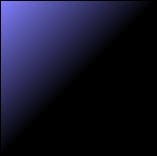This is a little bit more complicated example shown the implementation of diffuse and specular color.

```varying struct _v2f_ {
vec4 Position;
vec4 Normal;
} v2f;
```

Normal item is added for color computing. The main in vertex shader is not changed. The kernel add one line for normal computing.

```vec4 kernel(in mat4 Matrix, in vec4 Vertex)
{
v2f.Normal = normalize(gl_NormalMatrix * gl_Normal);
return (v2f.Position = Matrix * Vertex);
}
```

In vertex shader, gl_Position is the return of kernel function

```void main(void)
{
gl_Position=kernel(gl_ModelViewProjectionMatrix, gl_Vertex);
}
```

In fragment shader, the v2f variable is the same.

```void main( void )
{
vec4 normal = vec4(v2f.Normal, 0.0);
normal = normalize(normal);

vec4 light = vec4(1.0,1.0,3.0,1.0);
light = normalize(light);

vec4 eye = vec4(1.0, 1.0, 1.0, 0.0);
vec4 vhalf = normalize(light + eye);
```

transform normal from model-space to view-space, store normalized light vector, and calculate half angle vector. vec4(1.0, 1.0, 1.0, 0.0) is a vector constructor to initialize vector vec4 eye.

```  float diffuse = dot(normal, light);
float specular = dot(normal, vhalf);
specular = pow(specular, 32);
```

calculate diffuse and specular components with dot product and pow function.

```  vec4 diffuseMaterial = vec4(0.5, 0.5, 1.0, 1.0);
vec4 specularMaterial = vec4(0.5, 0.5, 1.0, 1.0);
```

set diffuse and specular material vector.

```  gl_FragColor = diffuse*diffuseMaterial + specular*specularMaterial;
}
```

add diffuse and specular components and output final vertex color.

## Draw TextureNow we are going to show how to add texture on a surface

```mat4 ModelViewProj;
mat4 ModelViewIT;
mat4 LightVec;
```

add new Texcoord component as texture coordinate with TEXCOORD0.

```varying struct _v2f_ {
vec4 Position;
vec2 Texcoord;
vec4 Color;
} v2f;
```

```void main( void )
{
gl_Position = gl_ModelViewProjectionMatrix * gl_Vertex;
v2f.Position = gl_Position;
v2f.Texcoord = vec2(gl_MultiTexCoord0);
v2f.Color = gl_Color;
}
```

```varying struct _v2f_ {
vec4 Position;
vec2 Texcoord;
vec4 Color;
} v2f;

uniform sampler2D tex0;
void main( void )
{
gl_FragColor = texture2D(tex0, v2f.Texcoord);
}
```

the code is the same as the above example except that the texture coord copy is added.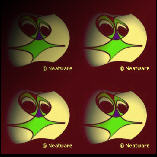Fragement shader completes the computing of pixels.

```float brightness;
uniform sampler2D tex0;
uniform sampler2D tex1;
```

brightness is the value to control the bright of light. Both tex0 and tex1 are the samplers of textures. Constant parameter brightness has a float type. sampler2D specifies a 2D texture unit.

```varying struct _v2f_ {
vec4 Position;
vec2 Texcoord0;
vec2 Texcoord1;
vec4 Color;
} v2f;
```

v2f declares a struct type that transfers data from vertex shader to fragment shader. It is the same as the struct in vertex shader. The vec4 Color and vec2 Texcoord* is for Color and Texture coordinates respectively.

```void main( void )
{
```

when you plan to access a texture you must use sampler* with an intrinsic function. A sampler can be used for multiple times.

```  vec4 color = texture2D(tex0, v2f.Texcoord0);
vec4 bump = texture2D(tex1, v2f.Texcoord1);
```

fetch texture color and bump coordinate for further computing of bump effect. Texture2D is a texture sampling intrinsic function of GLSL. It generates a vector from a texture sampler and a texture coordinate.

```  gl_FragColor = brightness * v2f.Color * color;
}
```

the code multiples brightness, v2f.Color and color to generate output RGBA color vector.

## Bump Mapping and Per-Pixel Lighting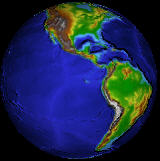This example demonstrates the use of vertex and fragment shaders for bump mapping. Bump mapping is a multitexture blending technique used to generate rough and bumpy surfaces. The data of Bump map (or normal map) are stored as a texture. Bump mapping includes an environment map and a bump data.

Per-pixel lighting provides an efficient and attractive method to render a surface. The standard lighting model consists of P for vertex position, N for unit normal of vertex, L for unit vector from light source to vertex, V for unit vector from vertex to view position, and R for unit reflection vector.

• diffuse lighting uses dot(N, L) as the color value.
• specular lighting (Phong) uses dot(R, V)n as a contribution of the color value.
• instead of using R, the Blinn simplification uses half vector between V and L.
```uniform sampler2D tex0;
uniform sampler2D tex1;
```

This is the bump mapping pixel shader with GLSL. v2f is the structure from vertex to pixel. Position is the vec4 vector, Diffuse is the diffuse color component, and Texcoord0, Texcooord1 are texture coordinates respectively.

```varying struct _v2f_ {
vec4 Position;
vec2 Texcoord0;
vec2 Texcoord1;
vec4 Diffuse;
} v2f;
```

Below is the main function of fragment shader.

```void main( void )
{
```

fetch base Color and Normal

```  vec4 Color = texture2D(tex0, v2f.Texcoord0);
vec4 Normal = texture2D(tex1, v2f.Texcoord1);
```

set final color

```  gl_FragColor = Color*dot(2.0*(Normal-0.5), 2.0*(v2f.Diffuse-0.5));
}
```

Following code is the vertex shader of GLSL.

```mat4 ModelWorld;
mat4 ModelViewProj;
vec4 vLight;
vec4 vEye;
vec4 vDiffuseMaterial;
vec4 vSpecularMaterial;
vec4 vAmbient;
float power;
```

global variables

```varying struct _v2f_ {
vec4 Position;
vec2 Texcoord0;
vec2 Texcoord1;
vec4 Diffuse;
} v2f;
```

the function cross below is defined as vector multiplication and/or as a swizzle operator.

```vec3 cross( vec3 a, vec3 b )
{
return vec3(a.y*b.z-a.z*b.y, a.z*b.x-a.x*b.z, a.z*b.y-a.y*b.z );
}
```

and its swizzle implementation

```vec3 cross(vec3 a, vec3 b)
{
return a.yzx*b.zxy - a.zxy*b.yzx;
}
```

declare attribute variable

```attribute vec3 Tangent;
```

define main function, get position

```void main( void )
{
```

transform normal from model-space to view-space

```  vec4 tangent = ModelWorld * vec4(Tangent.xyz, 0.0);
vec4 normal = ModelWorld * vec4(gl_NormalMatrix*gl_Normal.xyz,0.0);
vec3 binormal = cross(normal.xyz,tangent.xyz);
```

position in World

```  vec4 posWorld = ModelWorld * gl_Position;
```

get normalize light, eye vector, and half angle vector

```  vec4 light = normalize(posWorld-vLight);
vec4 eye = normalize(vEye-light);
vec4 vhalf = normalize(eye-vLight);
```

transform light and vhalf vectors to tangent space

```  vec3 L = vec3(dot(tangent,light),dot(binormal,light.xyz),
dot(normal,light));
vec3 H = vec3(dot(tangent,vhalf),dot(binormal,vhalf.xyz),
dot(normal, vhalf));
```

calculate diffuse and specular components

```  float diffuse = dot(normal, L);
float specular = pow(dot(normal, H), power);
```

combine diffuse and specular contributions and output final vertex color, set texture coordinates, and return output object.

```  gl_Position = gl_ModelViewProjectionMatrix * gl_Vertex;
v2f.Position = gl_Position;
v2f.Texcoord0 = vec2(gl_MultiTexCoord0);
v2f.Texcoord1 = vec2(gl_MultiTexCoord1);
v2f.Diffuse = 2.0*(diffuse*vDiffuseMaterial+ specular*vSpecularMaterial)
+ 0.5 + vAmbient;
}
```

Sobel Edge Filter in Image Processing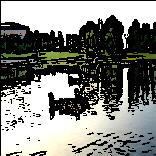This program shows the implementation of Sobel Edge Filter with a GLSL pixel shader. In the similar way we can implement many image filters in pixel shader.

```float Brightness;
uniform sampler2D tex0;

global variables
varying struct _v2f_ {
vec4 Position;
vec2 Texcoord;
vec4 Color;
} v2f;
```

```void main( void )
{
```

const specifies constants. c[NUM] is a vec2 constant array. Notes its initialization is convenience like C language. col[NUM] is a variable array of type vec3 with NUM elements. int i declares i as an integer.

```  const int NUM = 9;
const float threshold = 0.05;

const vec2 c[NUM] = {
vec2(-0.0078125, 0.0078125), vec2( 0.00 , 0.0078125),
vec2( 0.0078125, 0.0078125), vec2(-0.0078125, 0.00 ),
vec2( 0.0, 0.0 ), vec2( 0.0078125, 0.007 ),
vec2(-0.0078125,-0.0078125), vec2( 0.00 , -0.0078125),
vec2( 0.0078125,-0.0078125),
};

vec3 col[NUM];
int i;
```

stores the samples of texture to col array.

```  for (i=0; i < NUM; i++) {
col[i] = texture2D(tex0, a2f.Texcoord.xy + c[i]);
}
```

now we start to compute the luminance with dot product and store them in lum array.

```  vec3 rgb2lum = vec3(0.30, 0.59, 0.11);
float lum[NUM];
for (i = 0; i < NUM; i++) {
lum[i] = dot(col[i].xyz, rgb2lum);
}
```

Sobel filter computes new value at the central position by sum the weighted neighbors.

```  float x = lum+ lum+2*lum-lum-2*lum-lum;
float y = lum+2*lum+ lum-lum-2*lum-lum;
```

show the points which values are over the threshold and hide others. Final result is the product of col and edge detector value. Brightness adjusts the brightness of the image.

```  float edge =(x*x + y*y < threshold) ? 1.0 : 0.0;
```

final output

```  gl_FragColor = vec4(Brightness * col.xyz * edge.xxx, 1.0);
}
```

## References

HLSL Introduction